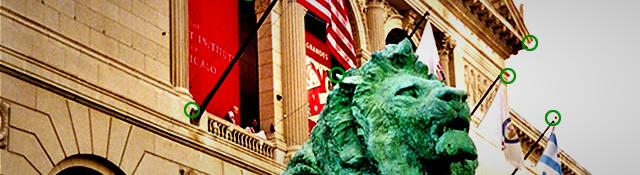## Corner Detection in OpenCV

OpenCV comes with both the Harris corner detector and the Shi-Tomasi corner detector. However, OpenCV uses the Shi-Tomasi corner detector unless you explicitly specify you want to use the other one. You can also get raw eigenvalues and eigenvectors for each pixel if you want, and process the values yourself. For now, we'll discuss the predefined functions.

## Good features to track

That's the name of the function! It's parameters look something like this:

```void cvGoodFeaturesToTrack(const CvArr* image,
CvArr* eig_image,
CvArr* temp_image,
CvPoint2D32f* corners,
int* corner_count,
double quality_level,
double min_distance,
int block_size=3,
int use_harris=0,
double k=0.04);
```

Lots of parameters. We'll go through each.

• image - Quite basic. This is the image you want to detect corners in. This image must be a grayscale image (8 bit, single channeled) or a 32-bit, single channeled image.
• eig_image - This is a temporary storage used by the function. Eigenvalues are calculated automatically and stored in this temporary image. This image should be a 32 bit, single channeled image. Also, it should be the same size as image. The data in this image can be useful (each point is the minimal eigenvalue associated with that pixel).
• temp_image - Another temporary storage that is used by the function. Unlike eig_image, this image is of no use after the function is over. It should be a 32 bit, single channeled image, with the same size as image.
• corners - Send a reference to a CvPoint2D32f array here. The function puts all "accepted" features into this array.
• corner_count - Send a reference to an integer here. You should set this integer to the maximum number of elements in the corners array before calling the function. After the function is over, it's value is set to the actual number of points in the array.
• quality_level - If you check the Shi-Tomasi criteria for selecting features, you'll see that it requires that the minimum eigenvalue should be above a certain predetermined value. quality_level is used to calculate this value. The threshold is calculated like this: λmin=quality_level * λmax Here λmin is the threshold, and λmax is the maximum eigenvalue through the image. Normal values for quality_level should be 0.01 or 0.1 (but always less than 1).
• min_distance - The minimum distance between two features. If two features or corners are closer than this distance, the function removes the weaker corner.
• mask - Again, quite basic. The function processes a pixel only if it is non-zero in the mask.
• block_size - It is better to consider a small region instead of a single point. This parameter lets you set the size of the window around a pixel.
• use_harris - (optional, default=0) Set this to a non-zero value if you want to use the Harris corner detector.
• k - (optional, default=0.04) If you decide to use the Harris method, you need to supply a 'k' to calculate a score for each pixel (check how a score is calculated in the Harris method)

## Summary

The cvGoodFeaturesToTrack function lets you find corners easily. By default, the function uses the Shi-Tomasi method, but you can use the Harris method as well.

#### Related posts#### Learning OpenCV: Computer Vision using the OpenCV Library

Utkarsh Sinha created AI Shack in 2010 and has since been working on computer vision and related fields. He is currently at Microsoft working on computer vision.